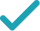Type a math problemSolve for xSteps Using the Quadratic Formula
Steps for Completing the Square
All equations of the form can be solved using the quadratic formula: . The quadratic formula gives two solutions, one when is addition and one when it is subtraction.
Subtract from both sides of the equation.
Subtracting from itself leaves .
This equation is in standard form: . Substitute for , for , and for in the quadratic formula, .
Square .
Multiply times .
The opposite of is .
Now solve the equation when is plus. Add to .
Now solve the equation when is minus. Subtract from .
The equation is now solved.
Solve for ySolution Steps
Swap sides so that all variable terms are on the left hand side.
Subtract from both sides.
Solve for x (complex solution)GraphGiving is as easy as 1, 2, 3
Get 1,000 points to donate to a school of your choice when you join Give With Bing
x^{2}-3x=y+3
All equations of the form ax^{2}+bx+c=0 can be solved using the quadratic formula: \frac{-b±\sqrt{b^{2}-4ac}}{2a}. The quadratic formula gives two solutions, one when ± is addition and one when it is subtraction.
x^{2}-3x-\left(y+3\right)=y+3-\left(y+3\right)
Subtract y+3 from both sides of the equation.
x^{2}-3x-\left(y+3\right)=0
Subtracting y+3 from itself leaves 0.
x=\frac{-\left(-3\right)±\sqrt{\left(-3\right)^{2}-4\left(-\left(y+3\right)\right)}}{2}
This equation is in standard form: ax^{2}+bx+c=0. Substitute 1 for a, -3 for b, and -\left(y+3\right) for c in the quadratic formula, \frac{-b±\sqrt{b^{2}-4ac}}{2a}.
x=\frac{-\left(-3\right)±\sqrt{9-4\left(-\left(y+3\right)\right)}}{2}
Square -3.
x=\frac{-\left(-3\right)±\sqrt{9+4y+12}}{2}
Multiply -4 times -\left(y+3\right).
x=\frac{-\left(-3\right)±\sqrt{4y+21}}{2}
x=\frac{3±\sqrt{4y+21}}{2}
The opposite of -3 is 3.
x=\frac{\sqrt{4y+21}+3}{2}
Now solve the equation x=\frac{3±\sqrt{4y+21}}{2} when ± is plus. Add 3 to \sqrt{21+4y}.
x=\frac{-\sqrt{4y+21}+3}{2}
Now solve the equation x=\frac{3±\sqrt{4y+21}}{2} when ± is minus. Subtract \sqrt{21+4y} from 3.
x=\frac{\sqrt{4y+21}+3}{2} x=\frac{-\sqrt{4y+21}+3}{2}
The equation is now solved.
x^{2}-3x=y+3
Quadratic equations such as this one can be solved by completing the square. In order to complete the square, the equation must first be in the form x^{2}+bx=c.
x^{2}-3x+\left(-\frac{3}{2}\right)^{2}=y+3+\left(-\frac{3}{2}\right)^{2}
Divide -3, the coefficient of the x term, by 2 to get -\frac{3}{2}=-1.5. Then add the square of -\frac{3}{2}=-1.5 to both sides of the equation. This step makes the left hand side of the equation a perfect square.
x^{2}-3x+\frac{9}{4}=y+3+\frac{9}{4}
Square -\frac{3}{2}=-1.5 by squaring both the numerator and the denominator of the fraction.
x^{2}-3x+\frac{9}{4}=y+\frac{21}{4}